Polar representation

Chapter 5 Class 11 Complex Numbers
Concept wise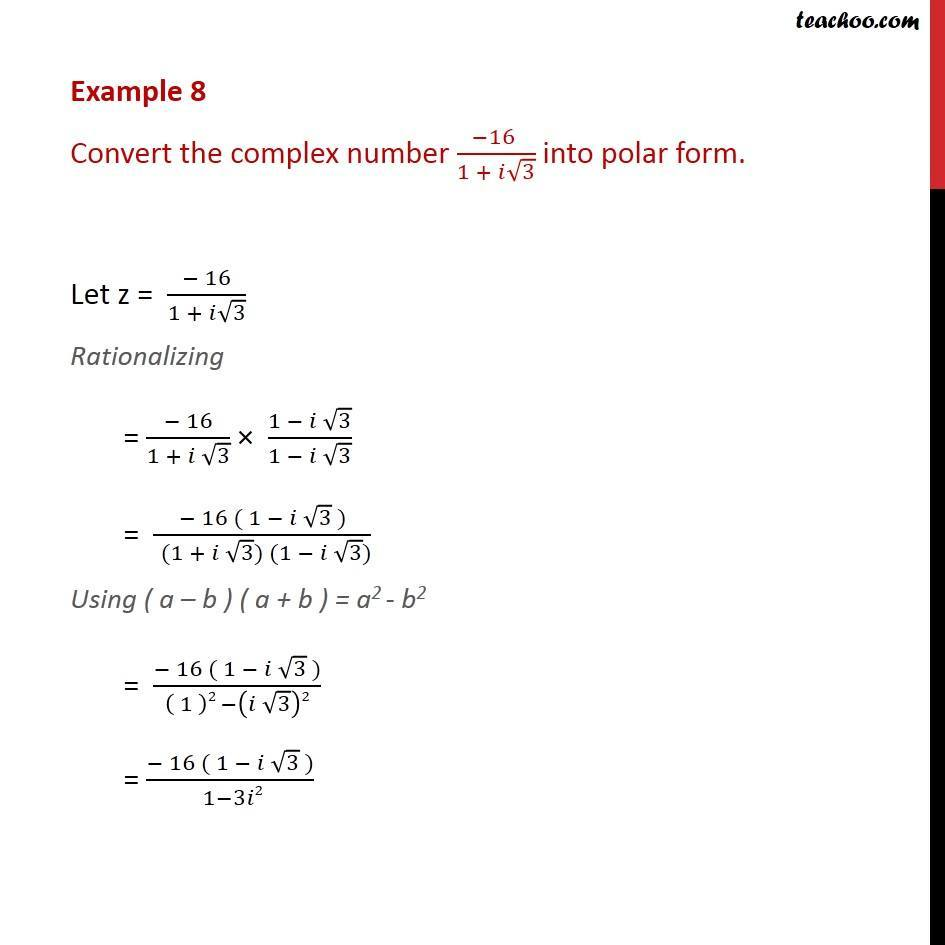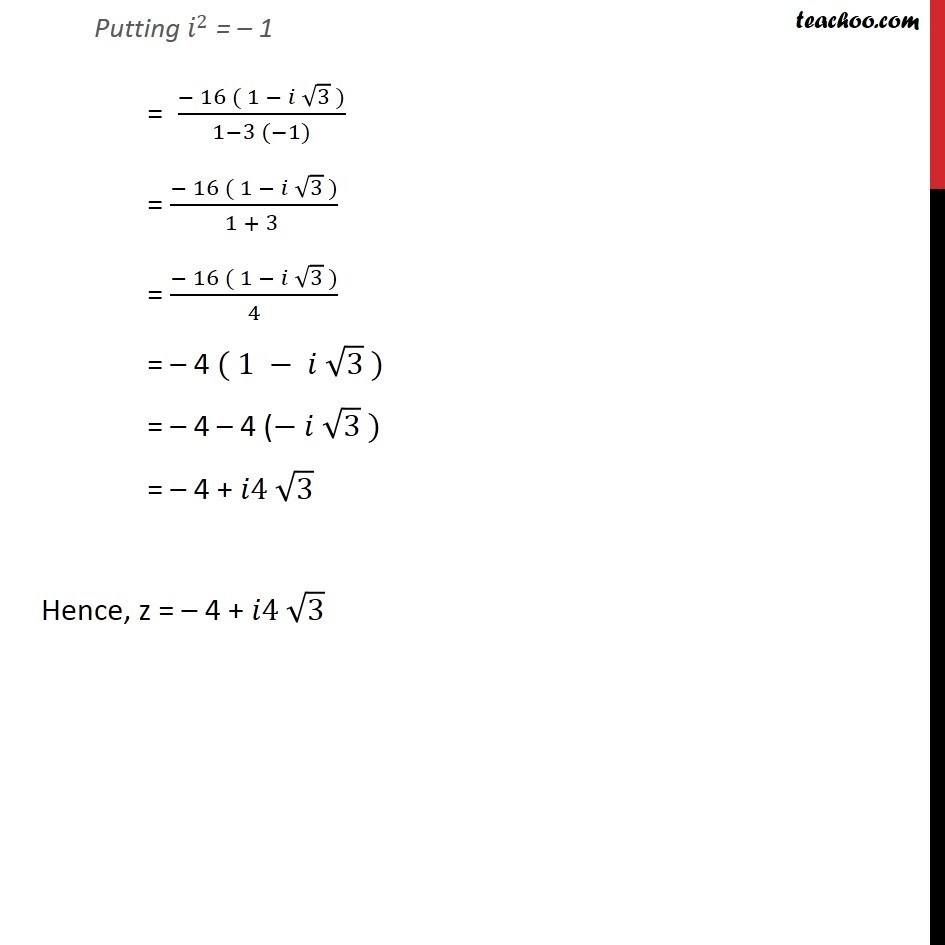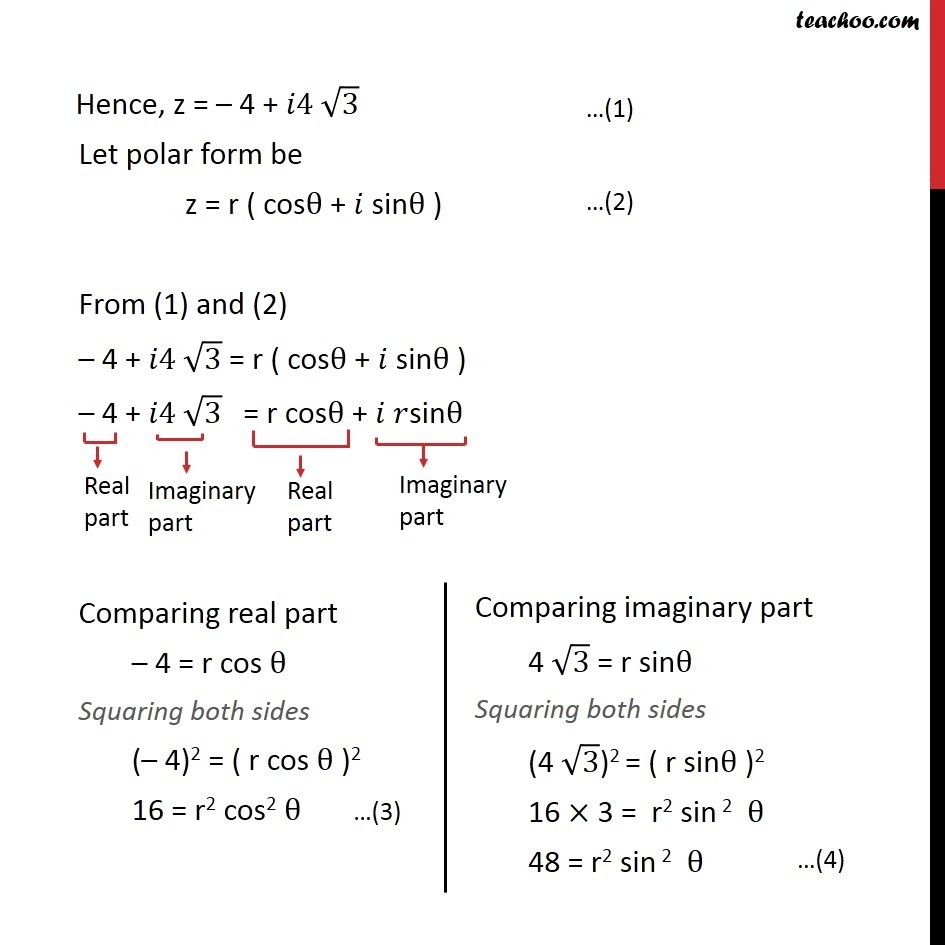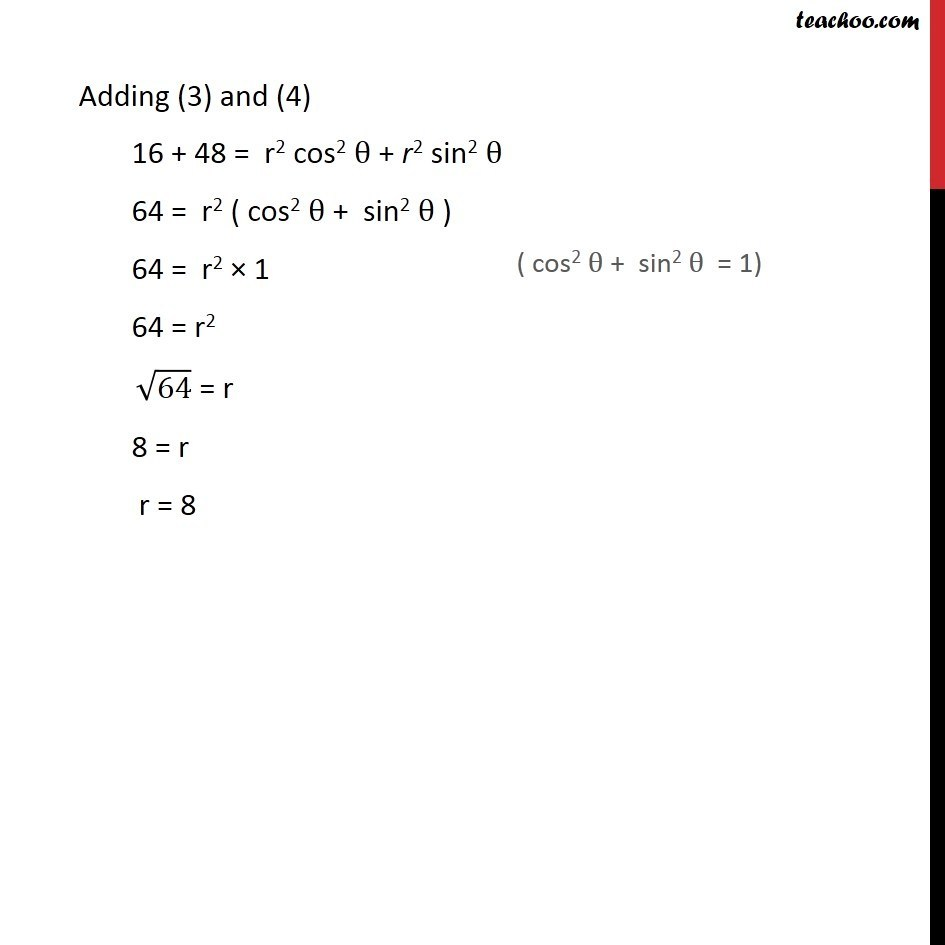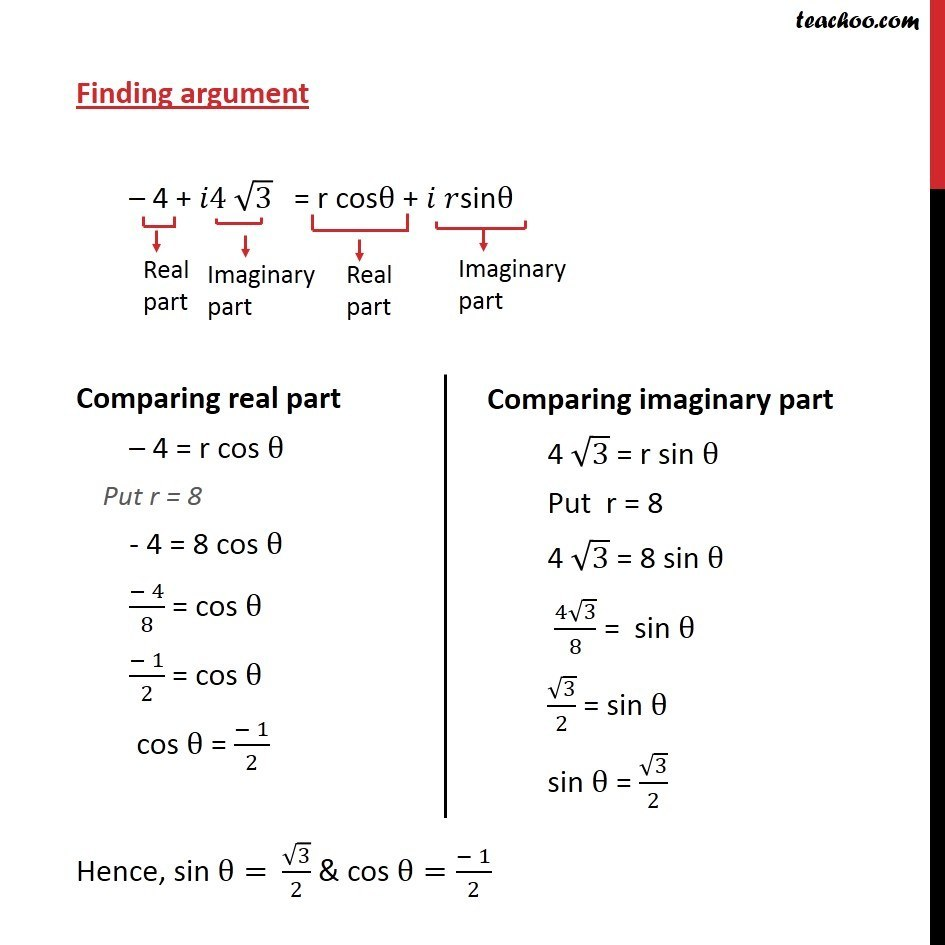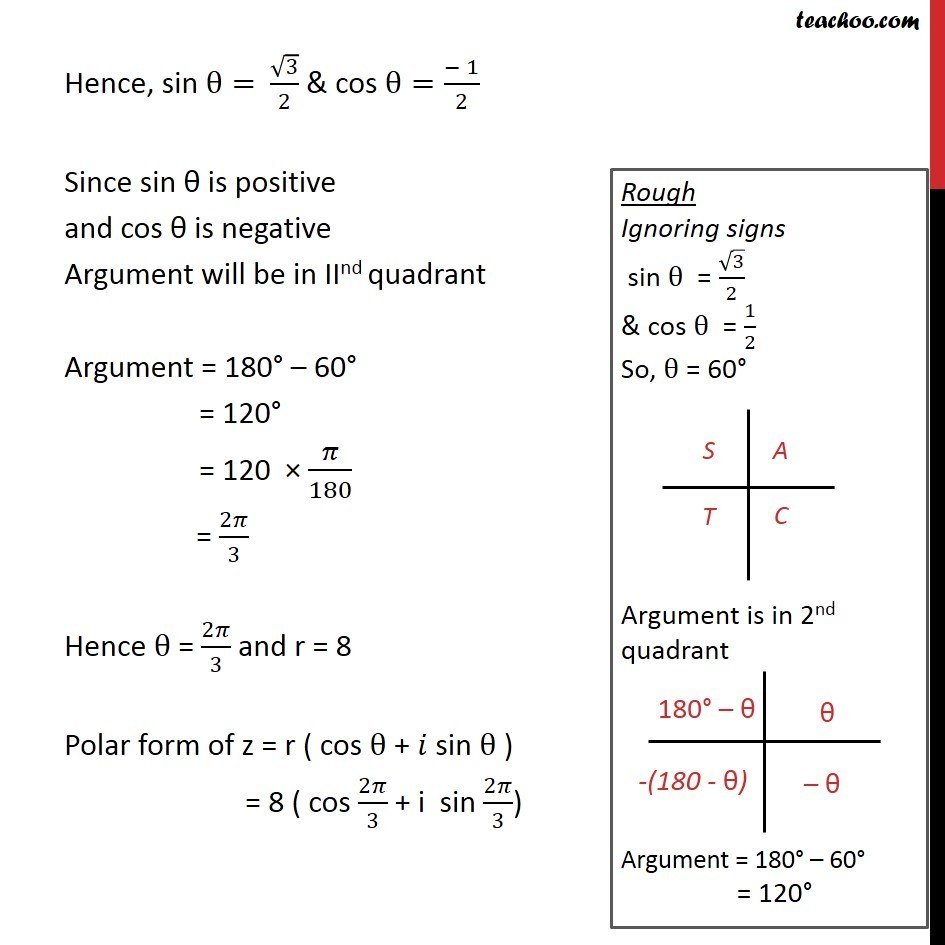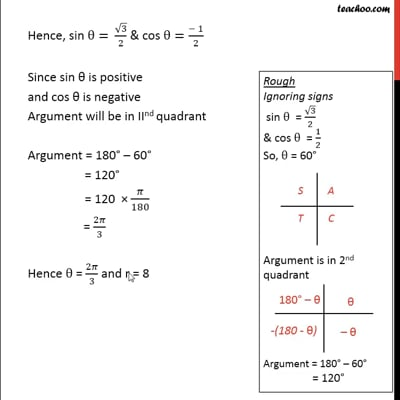This video is only available for Teachoo black users

Introducing your new favourite teacher - Teachoo Black, at only ₹83 per month

### Transcript

Example 8 Convert the complex number ( 16 )/(1 + 3) into polar form. Let z = ( 16)/(1 + 3) Rationalizing = ( 16)/(1 + 3) (1 3)/(1 3) = ( 16 ( 1 3 ))/(" " (1 + 3) (1 3)) Using ( a b ) ( a + b ) = a2 - b2 = ( 16 ( 1 3 ))/(( 1 )2 ( 3)2) = ( 16 ( 1 3 ))/( 1 3 2) Putting 2 = 1 = ( 16 ( 1 3 ))/(1 3 ( 1)) = ( 16 ( 1 3 ))/(1 + 3 ) = ( 16 ( 1 3 ))/4 = 4 ( 1 3 ) = 4 4 ( 3 ) = 4 + 4 3 Hence, z = 4 + 4 3 Hence, z = 4 + 4 3 Let polar form be z = r ( cos + sin ) From (1) and (2) 4 + 4 3 = r ( cos + sin ) 4 + 4 3 = r cos + sin Comparing real part 4 = r cos Squaring both sides ( 4)2 = ( r cos )2 16 = r2 cos2 Adding (3) and (4) 16 + 48 = r2 cos2 + r2 sin2 64 = r2 ( cos2 + sin2 ) 64 = r2 1 64 = r2 64 = r 8 = r r = 8 Finding argument 4 + 4 3 = r cos + sin Comparing real part 4 = r cos Put r = 8 - 4 = 8 cos ( 4)/8 = cos ( 1)/2 = cos cos = ( 1)/2 Hence, sin = 3/2 & cos =( 1)/2 Hence, sin = 3/2 & cos =( 1)/2 Since sin is positive and cos is negative Argument will be in IInd quadrant Argument = 180 60 = 120 = 120 /180 = 2 /3 Hence = 2 /3 and r = 8 Polar form of z = r ( cos + sin ) = 8 ( cos 2 /3 + i sin 2 /3)